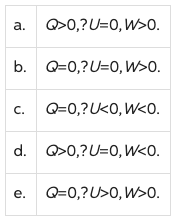# Problem: An ideal gas undergoes an isothermal expansion from state A to state B. In this process

###### FREE Expert Solution

The internal energy of a monoatomic gas is:

$\overline{){\mathbf{∆}}{\mathbf{U}}{\mathbf{=}}\frac{\mathbf{3}}{\mathbf{2}}{\mathbf{n}}{\mathbf{R}}{\mathbf{\left(}}{\mathbf{∆}}{\mathbf{T}}{\mathbf{\right)}}}$, where n is the number of moles, R is the universal gas constant, and ΔT is the change in temperature.

90% (445 ratings)###### Problem Details

An ideal gas undergoes an isothermal expansion from state A to state B. In this process